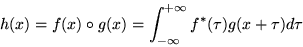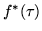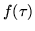Next: 13.4 Image Warping Up: 13.3 Convolutions Previous: 13.3.5.6.2 Vertical   Contents

### 13.3.6 Correlation and Feature Detection

The correlation operation is defined mathematically as:(16)

Theis the complex conjugate of, but since this section will discuss correlation for signals which only contain real values, substitute.

Correlation is useful for feature detection; applying correlation to an image that possibly contains a target feature and an image of that feature forms local maxima or pixel value spikes'' in candidate positions. This is useful in detecting letters on a page, or the position of armaments on a battlefield. Correlation can also be used to detect motion, such as the velocity of hurricanes in a satellite image or the jittering of an unsteady camera.

For two-dimensional discrete images, you may use Equation 15 to evaluate correlation.

The convolution extension (EXT_convolution) in OpenGL may be used to apply correlation to an image, but only for features no larger than the maximum convolution kernel size. For larger images or platforms which do not supply the convolution extension, use the accumulation buffer technique for convolution. (It is worth the effort to consider an alternative method, such as applying a multiplication in the frequency domain , if your feature and candidate images are very large.)

Once you have applied convolution, your application will need to find the spikes'' to determine where features have been detected. To aid this process, it may be useful to apply thresholding with a color table (SGI_color_table) to convert candidates pixels to one value and non-candidates to another.

One method used for finding features uses the following steps:

• Draw a small image containing just the feature to detect.
• Create a convolution filter containing that image.
• Transfer the image to the convolution filter using glCopyConvolutionFilter2DEXT().
• Draw your candidate image into the color buffers.
• Optionally configure a threshold for candidate pixels:
• Create a color table using glColorTableSGI().
• glEnableGL_ POST_CONVOLUTION_COLOR_TABLE_SGI(GL_ POST_CONVOLUTION_COLOR_TABLE_SGI).
• glEnableGL_ CONVOLUTION_2D_EXT(GL_ CONVOLUTION_2D_EXT)
• Apply pixel transfer to your candidate image using glCopyPixels().